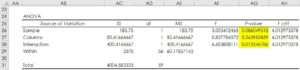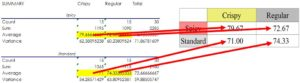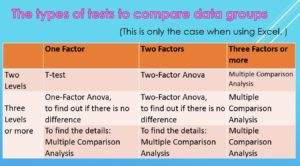Achieve Innovative Process Improvement＋Standardization by IT System with MS 365.# How to interpret the result of the Two-Factor Anova, Part 2: P-Value\ この記事を共有 /
How to interpret the result of the T...

This article is about how to interpret the results of Anova, including P-value, and connect it to our action. In order to understand P-value, you have to understand the concept of ‘Null Hypothesis’. This article explains the P-value and Null Hypothesis visually easy to understand manner.

(Duration: 8:01)

## What are the important points in the result of Two-Factor Anova?

Hi, this is Mike Negami, Lean Sigma, Black Belt.

In the last post, we went up to producing the result of Two-Factor Anova, with the Excel Data Analysis Tool. ⇒ “What’s ANOVA? (Analysis of Variance Basics) 【Excel Data Analysis Tools】 Part 1: Get the results quickly”

A quick recap from the last post, the data on the left in the image below is the score data that was rated by 60 people for 4 prototypes of fried chicken dishes in a restaurant.The data consists of the factor of ‘Texture’ such as “”Crispy”” and ‘Regular’ and another factor of ‘Seasoning’ such as ‘Spicy’ and ‘Standard’. I converted the data format from the flat format to the cross-tabulation format.

Then, in the Excel’s menu bar, click ‘Data’, ‘Data Analysis’, and ‘Anova: Two-Factor With Replication’.A lot of numbers came out. Of course, it’s better to understand the meaning of all the numbers, but today, I’ll give you the minimum information needed to use ANOVA in practice.

I added the same table to the right. The yellow highlighted parts are the most important. (See the image above.)

I added Excel functions in to that table to get the same results as in the table on the left, so that you can learn a bit deeper about the Anova results for yourself. You can download this Excel file from this video’s description below for your study, so please check what each value means later. ⇒”Download the Anova Sample File for study.”

## How to interpret the ‘P-value’ of Two-Factor Anova

The first three tables are not so difficult, but the last one may be challenging. It’s called the ‘Anova Table’. Of course, it’s good if you understand all numbers in the table, but at least understand this ‘P-Value’ well in Column AG.I talked about this ‘P-Value’ in the article about Regression Analysis. In more detail, P-value is the probability of the null hypothesis to occur. The ‘P’ in P-value stands for ‘Probability’. ⇒”Simple Regression Analysis Interpretation (Excel Data Analysis Tools)【Regression Analysis Series 2】”

The null hypothesis in Anova is “Assuming that the means of the data groups of each factor are equal””. Our null hypothesis would be “No difference in the scores of the four prototype dishes.”

Generally, if this percentage is 5% or less, you can reject a null hypothesis. In other words, discarding that “the averages are same” means “the differences between the averages of the data groups are significant”. With this in mind, let’s look at the our Anova table.

It says ‘Columns’ here in Cell AB27. That refers to the vertical direction, in our case, Crispy and Regular, in the columns. Their P-value is 0.36, which is 36% and more than 5%, so we cannot reject the null hypothesis. In other words, it means that whether it is Crispy or Regular, it doesn’t affect the scores.Next, it says ‘Sample’ above. That refers to the horizontal direction, Spicy and Standard. Their P-value is 0.08, which is 8%. This is lower possibility than the last one, but since it’s still higher than 5%, we cannot say that there were differences in scores between Spicy and Regular alone.

Lastly, let’s see the ‘Interaction’ below. Its P-value is 0.01, which is 1%. Since it’s below 5%, we can reject the null hypothesis. In other words, we cannot say that there are no differences, which means there are differences.

## How do we use the interpretation of Two-Factor ANOVA in practice?

Based on this, let’s look at the averages of each prototype’s scores. The Spicy Crispy Chicken has the highest score.We already knew that that Interaction has a significant difference in the scores, so we can conclude that it would be better to sell it in the form of Spicy Crispy rather than just making it Spicy or Crispy alone.

By the way, I borrowed the data from the web page of “”Fun statistics studied at a hamburger shop””. It’s in Japanese, and I put the site’s URL in this video’s description below.

## Limitations of Anova

In today’s example, that data has two factors, but each factor has only 2 levels, so we could judge the superiority or inferiority between levels by Anova and averages.

However, if there are three or more levels in one factor, and even if you could reject your null hypothesis, you can just say that “the averages of all data groups are not equal”, but you cannot identify which ‘Between Group’ has a significant difference.

Then, if there are more than two levels in one factor, can’t we use Anova? Yes, we can. You can use Anova when you want to find out if your data groups don’t have differences.

Now, what if there are more than two levels and you want to find out where is a difference?

Although Excel Data Analysis Tools don’t include this function, you can do a ‘Multiple Comparison Analysis’ using Excel functions. However, you need advanced knowledge of statistics such as multiplicity, etc. I may make a video about this if I receive request.

## The types of tests to compare data groups

This Anova content may be very confusing, so I summarized it in the table. This is only the case when using Excel.When there are only two data groups to compare, meaning there is only one factor with two levels, you can use T-test.

If there is one factor but three or more levels, you can use One-Factor Anova, but this is when you want to find out if there is no difference.

When there are two factors with 2 levels each, you can use Two-Factor Anova.

You can still use Anova when you want to check that there is no difference in the data groups with two factors with levels of 3 or more, but if you want to know the details in the data, you have to use Multiple Comparison Analysis.

This time I’ve simplified the very difficult contents considerably. Statistics experts, however, may have other opinions, so please put those in the comment section below, because I would like to deepen the discussion with you.

“See these other popular articles.”

### 最新記事